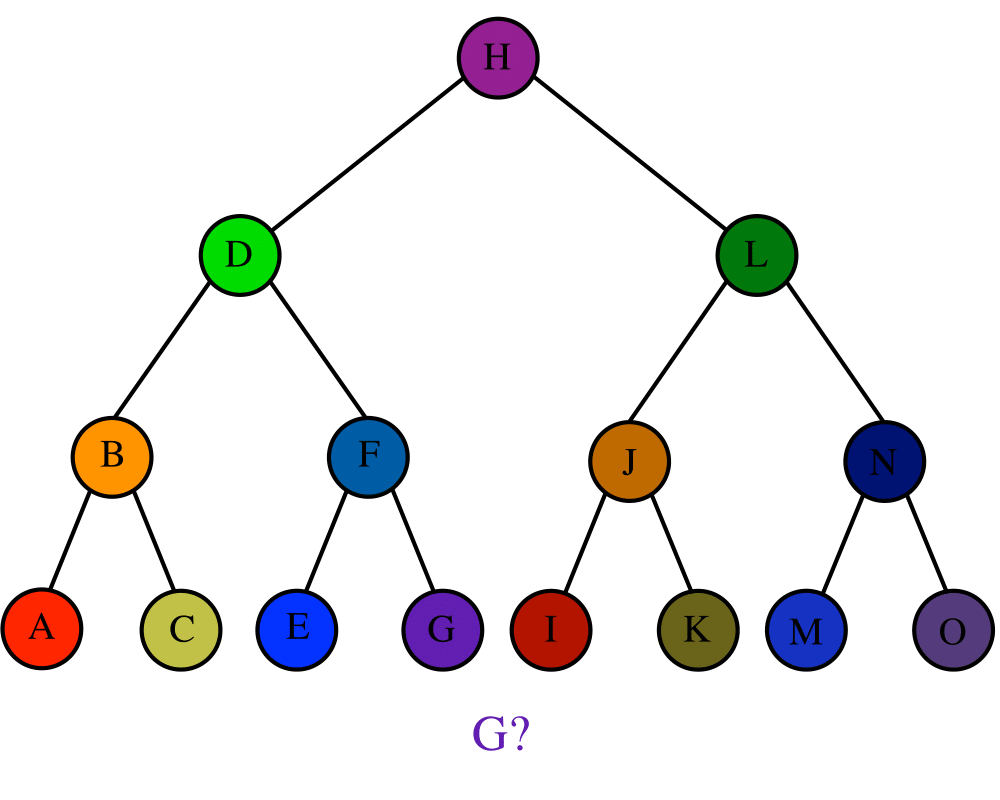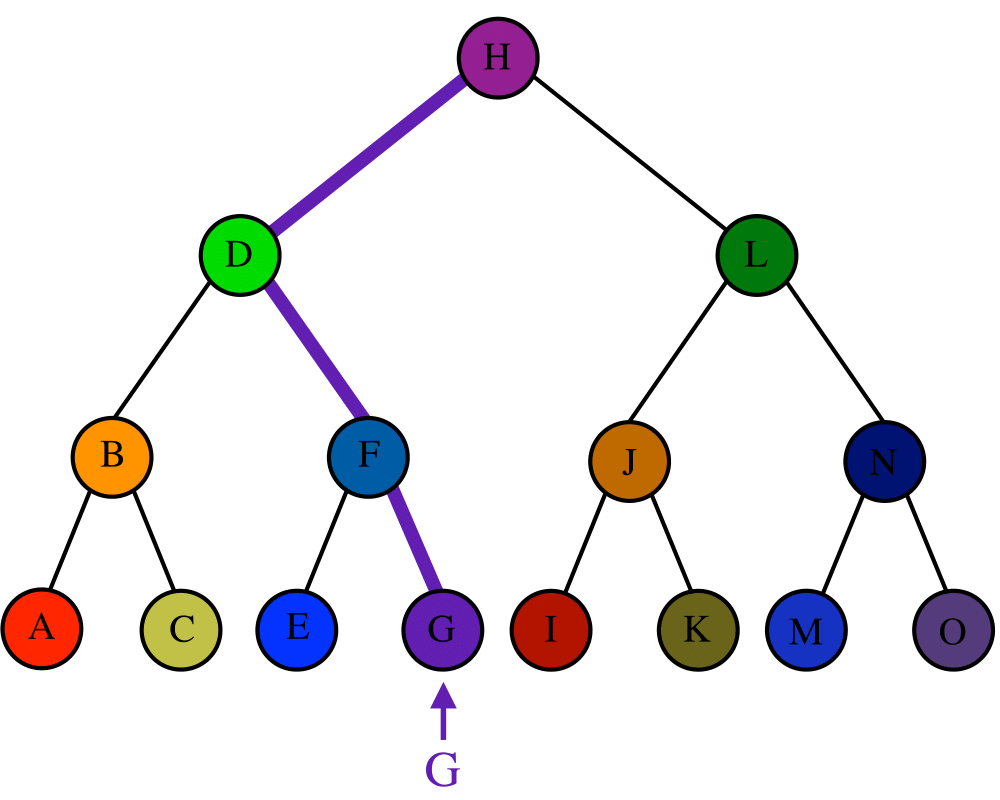# SearchingInputOutput

Input Description: A set $$S$$ of $$n$$ keys, a query key $$q$$.
Problem: Where is $$q$$ in $$S$$?

Excerpt from The Algorithm Design Manual: We treat searching here as a problem distinct from dictionaries because simpler and more efficient solutions emerge when our primary interest is static searching. These little data structures can yield large performance improvements when properly employed in an innermost loop. Also, several of the ideas from list and array searching, such as binary search and self-organization, apply to other problems and justify our attention.

There are two basic approaches to array searching: sequential search and binary search. Both are simple, yet have interesting and subtle variations. In sequential search, we simply start from the front of our list or array of keys and compare each successive item against the key until we find a match or reach the end. In binary search, we start with a sorted array of keys. To search for key $$q$$, we compare $$q$$ to the middle key $$S_{n/2}$$. If $$q$$ is before $$S_{n/2}$$, it must reside in the top half of our set; if not, it must reside in the bottom half of our set. By repeating this process on the correct half, we find the key in a total of $$\lceil \lg n \rceil$$ comparisons. This is a big win over the $$n/2$$ comparisons we expect with sequential search.

Go To Main Page